Courses

# Figure Matrix Quiz II, Non Verbal Reasoning

## 15 Questions MCQ Test Non Verbal Reasoning | Figure Matrix Quiz II, Non Verbal Reasoning

Description
This mock test of Figure Matrix Quiz II, Non Verbal Reasoning for LR helps you for every LR entrance exam. This contains 15 Multiple Choice Questions for LR Figure Matrix Quiz II, Non Verbal Reasoning (mcq) to study with solutions a complete question bank. The solved questions answers in this Figure Matrix Quiz II, Non Verbal Reasoning quiz give you a good mix of easy questions and tough questions. LR students definitely take this Figure Matrix Quiz II, Non Verbal Reasoning exercise for a better result in the exam. You can find other Figure Matrix Quiz II, Non Verbal Reasoning extra questions, long questions & short questions for LR on EduRev as well by searching above.
QUESTION: 1

### Directions to Solve In each of the following questions, find out which of the answer figures (1), (2), (3) and (4) completes the figure matrix ? Question - Select a suitable figure from the four alternatives that would complete the figure matrix.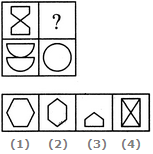Solution: The two parts of the first figure are rearranged and joined along the longer sides. The common side is then lost to form the second figure.
QUESTION: 2

### Directions to Solve In each of the following questions, find out which of the answer figures (1), (2), (3) and (4) completes the figure matrix ? Question - Select a suitable figure from the four alternatives that would complete the figure matrix.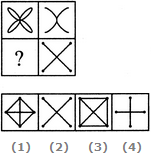Solution: The second figure is a part of the first figure (but is not exactly the same as the first figure).
QUESTION: 3

### Directions to Solve In each of the following questions, find out which of the answer figures (1), (2), (3) and (4) completes the figure matrix ? Question - Select a suitable figure from the four alternatives that would complete the figure matrix.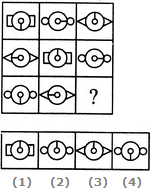Solution: In each row, the central part of the first figure rotates either 90o CW or 90o ACW to form the central part of the second figure and the central part of the first figure rotates through 180o to form the central part of the third figure. Also, in each row, there are 3 types of side elements - rectangles, circles and triangles.
QUESTION: 4

In each of the following questions, find out which of the answer figures (1), (2), (3) and (4) completes the figure matrix ?

Question -

Select a suitable figure from the four alternatives that would complete the figure matrix.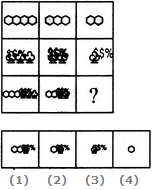Solution: In each column, the third figure (lowermost figure) contains one less number of hexagons as the first figure (uppermost figure) and the same number of trees as the second figure (middle figure).
QUESTION: 5

In each of the following questions, find out which of the answer figures (1), (2), (3) and (4) completes the figure matrix ?

Question -

Select a suitable figure from the four alternatives that would complete the figure matrix.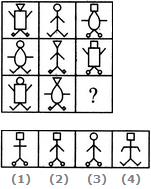Solution: There are 3 types of faces, 3 types of bodies, 3 types of hands and 3 types of legs, each of which is used only once in a single row. So, the features which have not been used in the first two figures of the third row would combine to produce the missing figure.
QUESTION: 6

In each of the following questions, find out which of the answer figures (1), (2), (3) and (4) completes the figure matrix ?

Question -

Select a suitable figure from the four alternatives that would complete the figure matrix.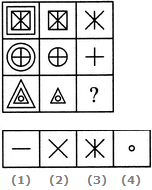Solution: In each row, the second figure is obtained by removing the outermost element of the first figure and the third figure is obtained by removing the outermost element of the second figure.
QUESTION: 7

In each of the following questions, find out which of the answer figures (1), (2), (3) and (4) completes the figure matrix ?

Question -

Select a suitable figure from the four alternatives that would complete the figure matrix.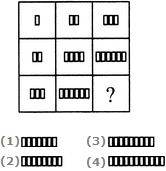Solution: In each row (as well as in each column), the number of rectangles in the second figure is two times the number of rectangles in the first figure and the number of rectangles in the third figure is three times the number of rectangles in the first figure.
QUESTION: 8

In each of the following questions, find out which of the answer figures (1), (2), (3) and (4) completes the figure matrix ?

Question -

Select a suitable figure from the four alternatives that would complete the figure matrix.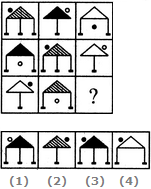Solution: There are 3 types of shadings in the triangles, 3 types of legs, 3 positions of circles, each of which is used only once in a single row. The circle is shaded in alternate figures.
QUESTION: 9

In each of the following questions, find out which of the answer figures (1), (2), (3) and (4) completes the figure matrix ?

Question -

Select a suitable figure from the four alternatives that would complete the figure matrix.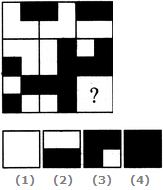Solution: In each row (as well as each column), the third figure is a combination of all the, elements of the first and the second figures
QUESTION: 10

In each of the following questions, find out which of the answer figures (1), (2), (3) and (4) completes the figure matrix ?

Question -

Select a suitable figure from the four alternatives that would complete the figure matrix.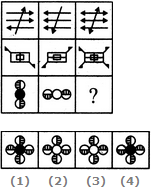Solution: In each row, the third figure is a combination of the first and the second figure.
QUESTION: 11

In each of the following questions, find out which of the answer figures (1), (2), (3) and (4) completes the figure matrix ?

Question -

Select a suitable figure from the four alternatives that would complete the figure matrix.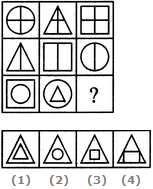Solution: In each row, the triangle follows the circle, the square follows the triangle and the circle follows the square. In case of the third row, the above rule exists for the inner as well as the outer elements.
QUESTION: 12

In each of the following questions, find out which of the answer figures (1), (2), (3) and (4) completes the figure matrix ?

Question -

Select a suitable figure from the four alternatives that would complete the figure matrix.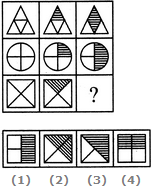Solution: In each row, the second figure is obtained by shading one of the four parts of the first figure and the third figure is obtained by shading two out of the four parts of the first figure.
QUESTION: 13

In each of the following questions, find out which of the answer figures (1), (2), (3) and (4) completes the figure matrix ?

Question -

Select a suitable figure from the four alternatives that would complete the figure matrix.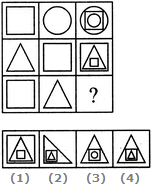Solution: In each row, the second figure forms the innermost and the outermost elements of the third figure and the first figure forms the middle element of the third figure.
QUESTION: 14

In each of the following questions, find out which of the answer figures (1), (2), (3) and (4) completes the figure matrix ?

Question -

Select a suitable figure from the four alternatives that would complete the figure matrix.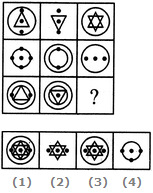Solution: The third figure in each row comprises of parts which are not common to the first two figures.
QUESTION: 15

In each of the following questions, find out which of the answer figures (1), (2), (3) and (4) completes the figure matrix ?

Question -

Select a suitable figure from the four alternatives that would complete the figure matrix.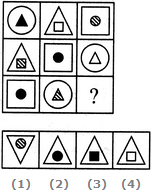Solution: In each row, there are three types of outer elements (circle, triangle and square), three types of inner elements (circle, triangle and square) and three types of shadings in the inner elements (black, white and lines).